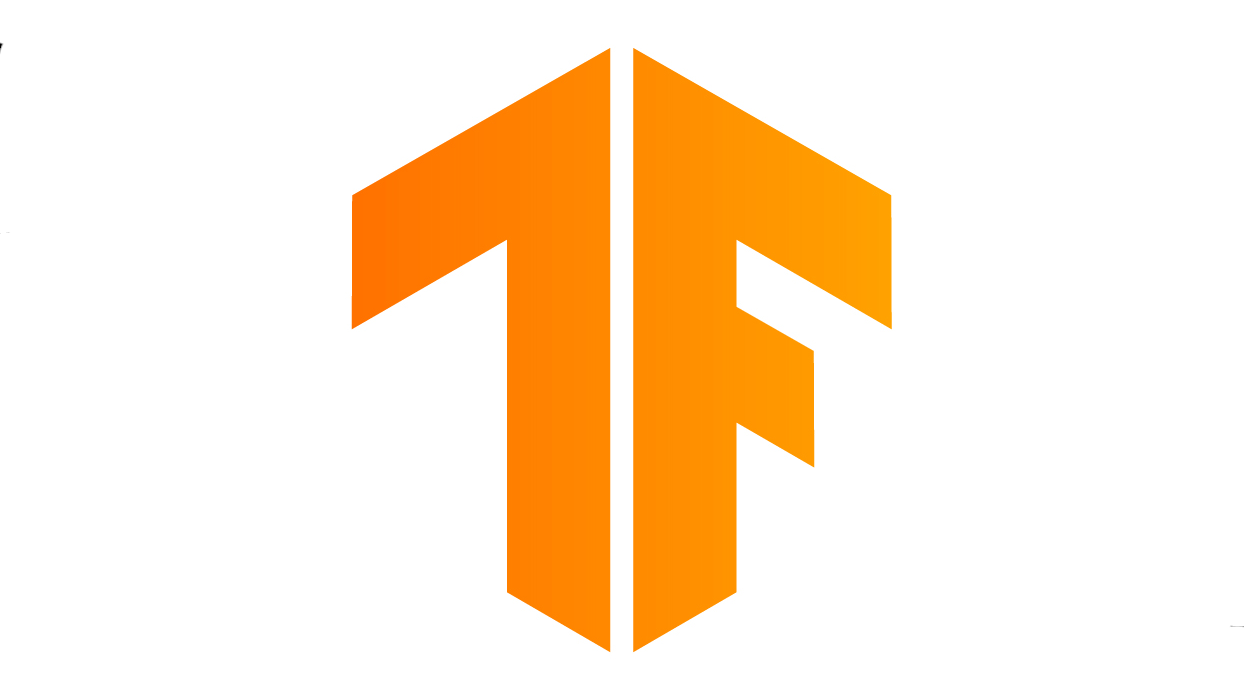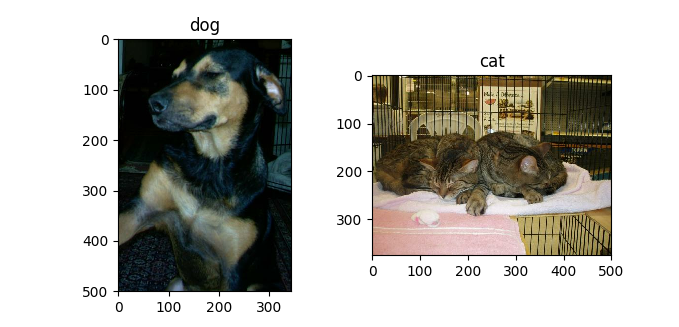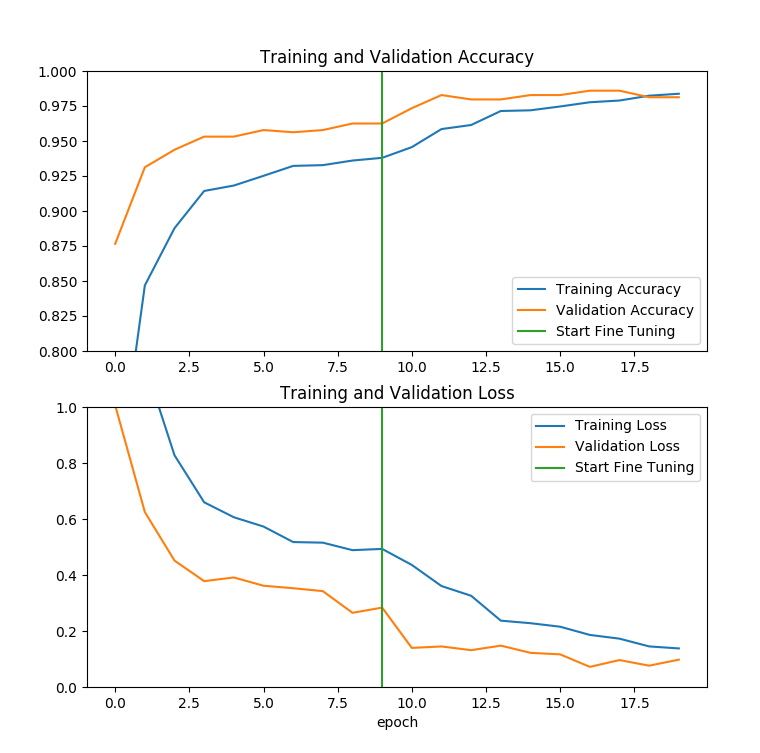# TensorFlow从1到2（九）#### 迁移学习基本概念

MobileNet V2是由谷歌在2018年初发布的一个视觉模型，在Keras中已经内置的并使用ImageNet完成了训练，可以直接拿来就用，这个我们在本系列第五篇中已经提过了。MobileNet V2有轻量和高效的特点。通过Inverted residual block结构，可以用较少的运算量得到较高的精度，很适合移动端的机器学习需求。论文在这里

#### 问题描述

MobileNet V2原本是识别图片中主题的名称。在ImageNet中，有大量的经过标注的照片，标注的信息也非常详细。比如我们熟悉的猫猫、狗狗，ImageNet并不简单的标注为cat或者dog，而是更详细的标注为加菲、德牧这样精确到具体品种的信息。

• 迁移学习并不是无限制、随意实现的。原有学习数据和数据的场景，同当前的问题，是有共同点、可借鉴可迁移的。
• 把cat、dog的具体品种忽略，简单的分成两类，并不能认为就是把问题简化了。要知道人工智能并不是人，举例来说，其实机器学习模型自己，并不知道“藏獒”跟“狗”之间有什么关系。在机器学习的模型中，会认为这是两个不同的分类。

#### 从简单开始，先展示一下识别

``````\$ pip3 install tfds-nightly
``````

``````#!/usr/bin/env python3

# 引入所使用到的扩展库
from __future__ import absolute_import, division, print_function
import os
import numpy as np
import matplotlib.pyplot as plt
import tensorflow as tf
import tensorflow_datasets as tfds

keras = tf.keras

# 载入训练数据，载入时按照80%:10%:10%的比例拆分为训练、验证、测试三个数据集
# 本程序只是演示识别图片，区分为三类并没有直接意义，但下面的程序训练模型会使用到
SPLIT_WEIGHTS = (8, 1, 1)
splits = tfds.Split.TRAIN.subsplit(weighted=SPLIT_WEIGHTS)
'cats_vs_dogs', split=list(splits),
with_info=True, as_supervised=True)
# 图片标注

# 显示头两幅图片
i = 1
plt.figure('Dog & Cat', figsize=(8, 4))
for image, label in raw_train.take(2):
plt.subplot(1, 2, i)
plt.imshow(image)
plt.title(get_label_name(label))
i += 1
plt.show()

# 所有图片转为224x224的尺寸，适应模型的要求
IMG_SIZE = 224
def format_example(image, label):
image = tf.cast(image, tf.float32)
image = (image/127.5) - 1
image = tf.image.resize(image, (IMG_SIZE, IMG_SIZE))
return image, label
train = raw_train.map(format_example)

# 载入模型，第一次执行会下载h5文件
# 预测和迁移学习所用的模型并不完全相同，所以会下载两次
test_model = tf.keras.applications.MobileNetV2(weights='imagenet')

# 预测头两张照片并显示结果
for image, _ in train.take(2):
img = np.expand_dims(image, axis=0)
predict_class = test_model.predict(img)
desc = tf.keras.applications.mobilenet_v2.decode_predictions(predict_class, top=3)
print(desc)
``````

``````\$ ./cats_dogs_1predict.py
[('n02089078', 'black-and-tan_coonhound', 0.46141574), ('n02105412', 'kelpie', 0.15314996), ('n02106550', 'Rottweiler', 0.092713624)]
[('n02123045', 'tabby', 0.29928064), ('n02123159', 'tiger_cat', 0.08147916), ('n02096177', 'cairn', 0.047330838)]
``````#### 迁移学习改造

MobileNet V2模型默认是将图片分类到1000类，每一类都有各自的标注。因为本问题分类只有两类，所以在代码上，我们构建模型的时候增加include_top=False参数，表示我们不需要原有模型中最后的神经网络层（分类到1000类），以便我们增加自己的输出层。当然这样在第一次执行程序的时候，需要重新下载另外一个不包含top层的h5模型数据文件。

``````#!/usr/bin/env python3

# 引入所使用到的扩展库
from __future__ import absolute_import, division, print_function
import os
import numpy as np
import matplotlib.pyplot as plt
import tensorflow as tf
import tensorflow_datasets as tfds

keras = tf.keras

# 载入训练数据，载入时按照80%:10%:10%的比例拆分为训练、验证、测试三个数据集
SPLIT_WEIGHTS = (8, 1, 1)
splits = tfds.Split.TRAIN.subsplit(weighted=SPLIT_WEIGHTS)
'cats_vs_dogs', split=list(splits),
with_info=True, as_supervised=True)

# cat/dog两类图片，复杂度要低于1000类的图片，所以分辨率可以略低，这样也能节省训练时间
# 所有图片重新调整为160x160点阵
IMG_SIZE = 160
def format_example(image, label):
image = tf.cast(image, tf.float32)
# 数据规范化为-1到+1的浮点数
image = (image/127.5) - 1
image = tf.image.resize(image, (IMG_SIZE, IMG_SIZE))
return image, label

train = raw_train.map(format_example)
validation = raw_validation.map(format_example)
test = raw_test.map(format_example)

BATCH_SIZE = 32
SHUFFLE_BUFFER_SIZE = 1000
train_batches = train.shuffle(SHUFFLE_BUFFER_SIZE).batch(BATCH_SIZE)
validation_batches = validation.batch(BATCH_SIZE)
test_batches = test.batch(BATCH_SIZE)

# 输入形状就是160x160x3,最后3为RGB3字节色
IMG_SHAPE = (IMG_SIZE, IMG_SIZE, 3)
base_model = tf.keras.applications.MobileNetV2(input_shape=IMG_SHAPE,
include_top=False,  # 使用不包含原有1000类输出层的模型
weights='imagenet')
# 设置基础模型：MobileNetV2的各项权重参数不会被训练所更改
base_model.trainable = False
# 输出模型汇总信息
base_model.summary()

# 增加输出池化层
global_average_layer = tf.keras.layers.GlobalAveragePooling2D()

# 输出层
prediction_layer = keras.layers.Dense(1)
# 定义最终完整的模型
model = tf.keras.Sequential([
base_model,
global_average_layer,
prediction_layer
])
# 学习梯度
base_learning_rate = 0.0001
# 编译模型
model.compile(optimizer=tf.keras.optimizers.RMSprop(lr=base_learning_rate),
loss='binary_crossentropy',
metrics=['accuracy'])

# 各部分数据数量
num_train, num_val, num_test = (
for weight in SPLIT_WEIGHTS
)
# 迭代次数
initial_epochs = 10
steps_per_epoch = round(num_train)//BATCH_SIZE
# 验证和评估次数
validation_steps = 20

# 显示一下未经训练的初始模型评估结果
loss0, accuracy0 = model.evaluate(test_batches, steps=validation_steps)
print("initial loss: {:.2f}".format(loss0))
print("initial accuracy: {:.2f}".format(accuracy0))

# 训练
history = model.fit(train_batches.repeat(),
epochs=initial_epochs,
steps_per_epoch=steps_per_epoch,
validation_data=validation_batches.repeat(),
validation_steps=validation_steps)
# 评估
loss0, accuracy0 = model.evaluate(test_batches, steps=validation_steps)
print("Train1ed loss: {:.2f}".format(loss0))
print("Train1ed accuracy: {:.2f}".format(accuracy0))
``````

``````Train1ed loss: 0.38
Train1ed accuracy: 0.95
``````

#### 模型优化

``````	...继续前面的代码最后输入...
# 打开允许修改基础模型的权重参数
base_model.trainable = True
# 仍然锁死前100层的权重不允许修改
fine_tune_at = 100
# 冻结fine_tune_at之前的层
for layer in base_model.layers[:fine_tune_at]:
layer.trainable = False
# 重新编译模型
model.compile(loss='binary_crossentropy',
optimizer=tf.keras.optimizers.RMSprop(lr=base_learning_rate/10),
metrics=['accuracy'])
# 输出模型的概况，注意观察有些层被锁定，有些层可以更改
model.summary()
# 在前次训练的基础上再训练10次
fine_tune_epochs = 10
total_epochs = initial_epochs + fine_tune_epochs
# 训练模型
history_fine = model.fit(train_batches.repeat(),
steps_per_epoch=steps_per_epoch,
epochs=total_epochs,
initial_epoch=initial_epochs,
validation_data=validation_batches.repeat(),
validation_steps=validation_steps)
# 评估新模型
loss0, accuracy0 = model.evaluate(test_batches, steps=validation_steps)
print("final loss: {:.2f}".format(loss0))
print("final accuracy: {:.2f}".format(accuracy0))

``````

``````Model: "sequential"
_________________________________________________________________
Layer (type)                 Output Shape              Param #
=================================================================
mobilenetv2_1.00_160 (Model) (None, 5, 5, 1280)        2257984
_________________________________________________________________
global_average_pooling2d (Gl (None, 1280)              0
_________________________________________________________________
dense (Dense)                (None, 1)                 1281
=================================================================
Total params: 2,259,265
Trainable params: 1,863,873
Non-trainable params: 395,392
``````

Non-trainable params这一部分，就是指我们锁定不参与新数据训练的参数数量。如果你注意看前面一部分base_model.summary()的输出，所有的参数都被锁定不参与训练。

``````final loss: 0.23
final accuracy: 0.97
``````

``````	...继续前面的代码最后输入...
# 优化前训练迭代数据
acc = history.history['accuracy']
val_acc = history.history['val_accuracy']

loss = history.history['loss']
val_loss = history.history['val_loss']

# 优化后训练迭代数据
acc += history_fine.history['accuracy']
val_acc += history_fine.history['val_accuracy']

loss += history_fine.history['loss']
val_loss += history_fine.history['val_loss']

# 使用训练数据绘图
plt.figure(figsize=(8, 8))
plt.subplot(2, 1, 1)
plt.plot(acc, label='Training Accuracy')
plt.plot(val_acc, label='Validation Accuracy')
plt.ylim([0.8, 1])
plt.plot([initial_epochs-1,initial_epochs-1],
plt.ylim(), label='Start Fine Tuning')
plt.legend(loc='lower right')
plt.title('Training and Validation Accuracy')

plt.subplot(2, 1, 2)
plt.plot(loss, label='Training Loss')
plt.plot(val_loss, label='Validation Loss')
plt.ylim([0, 1.0])
plt.plot([initial_epochs-1,initial_epochs-1],
plt.ylim(), label='Start Fine Tuning')
plt.legend(loc='upper right')
plt.title('Training and Validation Loss')
plt.xlabel('epoch')
plt.show()
``````（待续…）